Latest Banking jobs   »

# Quantitative Aptitude Quiz For IBPS RRB PO/Clerk Prelims 2022- 26th July

Directions (1-5): In the following questions, calculate quantity I and quantity II, compare them and answer
(a) If quantity I > quantity II
(b) If quantity I < quantity II
(c) If quantity I ≥ quantity II
(d) if quantity I ≤ quantity II
(e) if quantity I = quantity II or no relation can be established

Q1. Quantity I: If the printer is sold at a profit of 10% instead of 10% of loss, the shopkeeper gets Rs.360 more, then find the cost price of printer (in Rs)?
Quantity II: A seller marked up the price of a bag by 25% and gives a discount of 6⅔%. Find selling price of the bag (in Rs), if the cost price of the bag is Rs 1200?

Q2. Quantity I, x:x²-16x+48=0
Quantity II, y:y²-13y+12=0

Q3. Quantity I: Pipe A alone and pipe B alone can fill a tank in 40 minutes and 1 hour respectively. But it is found that there is leakage in the tank, due to which pipes takes 6 minutes extra to fill the tank when both pipes are open together. Find time taken by leakage to empty the filled tank alone (in min)?
Quantity II: 108 minutes

Q4. Quantity I, x: x²+7x+12=0
Quantity II, y: y²+13y+36=0

Q5. Quantity I: If the total time taken by man to cover the whole distance is 4 hours, in which he covers half of distance at a speed of 10 kmph and remaining half at a speed of 15 kmph. Then find distance traveled by man (in km)?
Quantity II: 48 km

Directions (6-10): There are two statements i.e. I and II given in the following questions, read the information of each statement and give answer as follows
(a) Statement (I) alone is sufficient to answer the question but statement (II) alone is not sufficient to answer the questions.
(b) Statement (II) alone is sufficient to answer the question but statement (I) alone is not sufficient to answer the question.
(c) Both the statements taken together are necessary to answer the questions, but neither of the statements alone is sufficient to answer the question.
(d) Either statement (I) or statement (II) by itself is sufficient to answer the question.
(e) Statements (I) and (II) taken together are not sufficient to answer the question.

Q6. Find the marked price of table?
I. A shopkeeper purchases two tables for Rs. 1600 and earns Rs. 100 on each table after giving discount of 10% on each table.
II. A shopkeeper gives 20% discount on mark price of a table and discount on chair is Rs. 700 less than cost price of a table.

Q7. Find total distance traveled by boat in upstream and in downstream together?
I. A boat takes 8 hours to cover a distance in upstream and 3 hours to cover the same distance in downstream.
II. The speed of boat in still water is 8 kmph and speed of stream is 5 kmph.

Q8. Find the length of train A?
I. The length of train B is 100 meter and sum of speed of train A and train B is 126 km/h.
II Train B is faster than train A and crosses train A in 32 sec while moving in same direction.

Q9. Find the present age of Sheetal?
I. The average of present ages of Deeksha, Sheetal and Aryan is 30 years and ratio of their present ages is 4: 5: 3 respectively.
II. Aryan is 2.5 years older than Sheetal. Five years ago, age of Deeksha was twice of age of Sheetal.

Q10. Find the compound interest earned in two years?
I. A sum becomes Rs.720 in 2 years when rate is compounded half yearly.
II. Interest earned on third year is Rs.288 when rate is compounded annually.

Directions (11-15): In the following questions, calculate quantity I and quantity II, compare them and answer
(a) If quantity I > quantity II
(b) If quantity I < quantity II
(c) If quantity I ≥ quantity II
(d) if quantity I ≤ quantity II
(e) if quantity I = quantity II or no relation can be established

Q11. Quantity I: 42 months
Quantity II: Brijesh borrows Rs.40,000 from a bank at SI at the rate of 10% per annum. He settled up the amount by giving Rs.52,000 to bank after some time. Find the time period after which he clears his debt? (in months)

Q12. Quantity I: Divya and Siya working together can complete a piece of work in 15 days. If Divya, Siya and Anil work together they complete the same work in 12 days. The efficiency of Siya is 100% more than Anil. Find the time taken (in days) by Siya to complete the work alone.
Quantity II: 32 days

Q13. Quantity I, x: x²-8x+15=0
Quantity II, y: y²-11y+30=0

Q14. A, B and C starts a business with investment of Rs.2000, Rs.2500, and Rs.5000, After 6 months, A invests Rs.1250 more while C withdraws all his money and after 8 months of business B invests Rs.1000 more. In the end of the year total profit earned by them is Rs.19100.
Quantity I: Total profit of A and B together.
Quantity II: Total profit of B and C together.

Q15. Quantity I: In how many different ways letters of the word INDIANA can be arranged.
Quantity II: in how many different ways letters of the word PARASITE can be arranged.

Solutions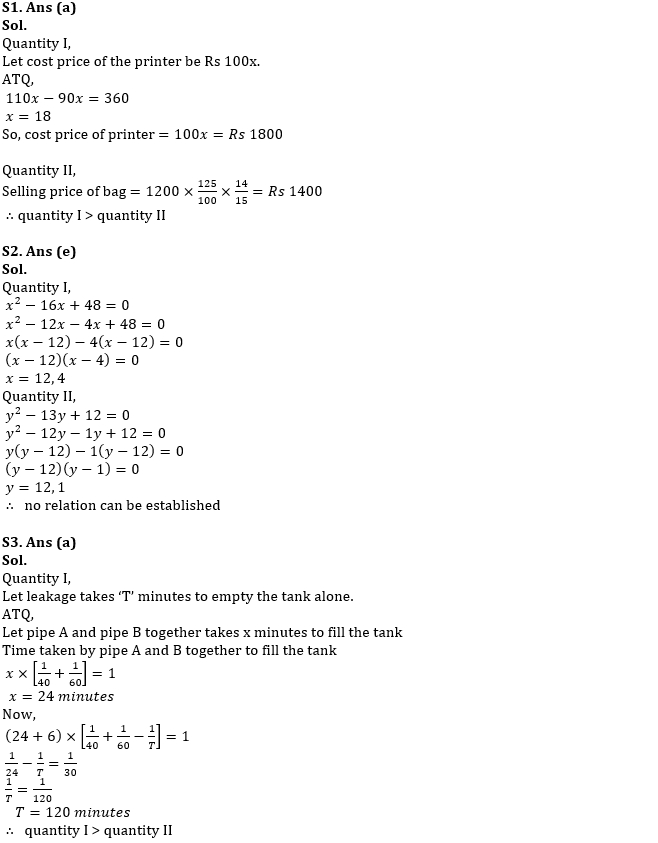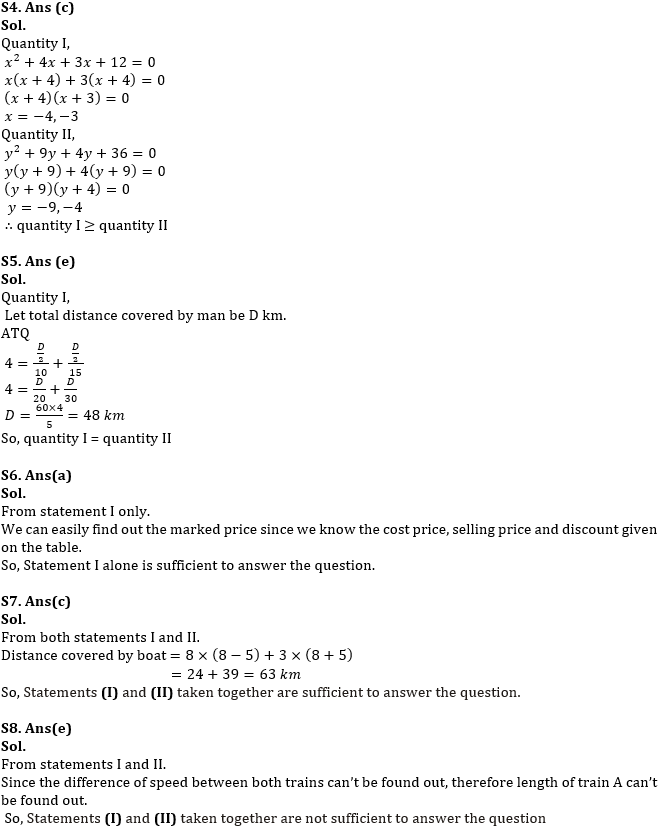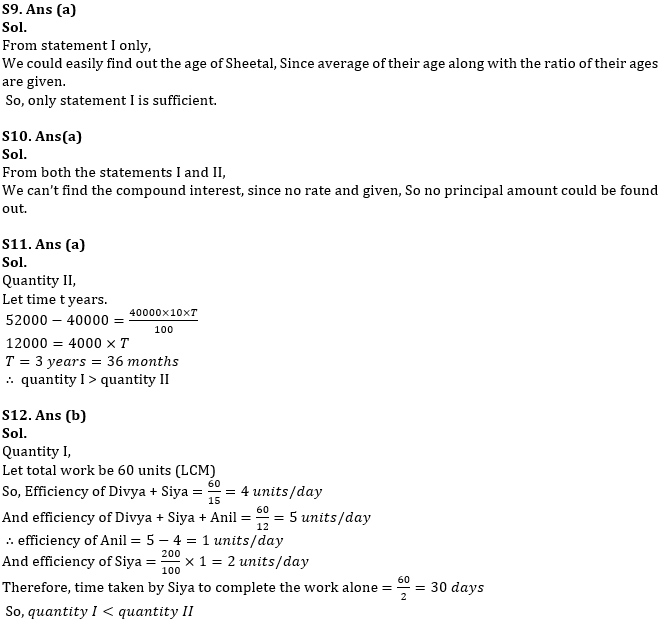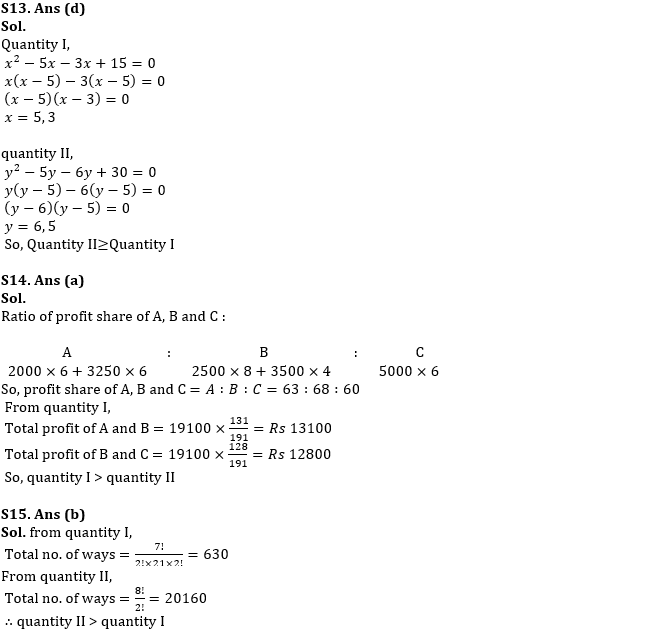#### Congratulations!Incorrect details? Fill the form again here

•Reasoning Ability Quiz For IBPS RRB PO C...
•Quantitative Aptitude Quiz For IBPS RRB ...
•Reasoning Ability Quiz For IBPS RRB PO C...
•Quantitative Aptitude Quiz For IBPS RRB ...
•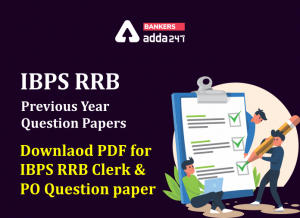IBPS RRB Previous Year Question Paper PD...
•Quantitative Aptitude Quiz For IBPS RRB ...